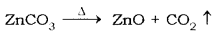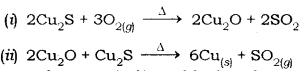# (a) Write the steps involved in the extraction of pure metals

(a) Write the steps involved in the extraction of pure metals in the middle of the activity series from their carbonate ores.
(b) How is copper extracted form its sulphide ore? Explain the various steps supported by chemical equations. Draw labelled diagram for the electrolytic refining of copper.

(a) Let us consider the case of Zn metal extraction from its carbonate ore.
Steps involved are:

1. The ore ZnCO3 is first concentrated by gravity separation method.
2. The ore is calcinated absence of it gets converted to oxide3. The oxide is reduced by coke
ZnO + C → Zn + CO
4. The impure Zn thus obtained can be purified by electrolysis.

(b) Copper from its sulphide ore can be extracted simply by heating in air.
The steps involved are